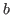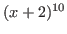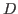: Package files in the : kan/examples : About this document   目次

# Getting started

To start the system, type in sm1. To quit the system, type in quit. You can make a program run in kan/sm1 by the operator

```        (filename) run  ;
```
or
```        \$filename\$ run  ;
```
The two expressions ` \$xyz\$ ` and (xyz) have the same meaning; they means the string xyz. The pair of brackets generates a string object. The dollar sign is used for a compatibility to kan/sm1 Version 1.x.

There are three groups of functions. The first group is those of primitive operators. They are functions written in C. The second group is those of macro operators. They are functions written in sm1 language and automatically loaded when the system starts. The third group is those of macro operators defined in the library files in lib/ directory. These operators provide a user friendly interfaces of computing characteristic ideal, holonomic rank,-function, annihilating ideal, hypergeometric differential operators, restrictions, de Rham cohomology groups. You can get a list of primitive operators and macros by ? and ?? respectively. To see the usage of a macro, type in (macro name) usage ; . Note that you need a space before ;. All tokens should be separated by the space or special characters ` ( ) [ ] { } \$ % `. The help message usually provides examples. For example, the line (add) usage present the example ` Example: 2 3 add :: ==> 5` You may try the input line 2 3 add :: and will get the output 5. All printable characters except the special characters ` ( ) [ ] { } \$ % ` can be a part of a name of a macro or primitive operator. For example, :: is a name of macro which outputs the top of the stack and the prompt.

kan/sm1 is a stack machine. Any object that has been input is put on the top of the stack. Any operator picks up objects from the stack, executes computations and puts results on the stack. For example, the primitive operator print picks up one object from the stack and print it to the screen. If you type in (Hello World) print, then the string ``Hello World'' is put on the stack and the operator print picks up the string and print it. The macro message works like print and outputs the newline. The macro :: is similar to message, but it also outputs the newline and the prompt; it picks up one object from the stack, print the object to the screen and output the prompt sm1>. For example, when you type in

```    (Hello World) ::
```
you get
```Hello World
sm1>
```
We introduce two more useful stack operators.
pop     Remove the top element from the stack.
pstack     Print the contents of the entire stack.
You can use kan/sm1 as a reverse Polish calculator; try the following lines.
```     11 4 mul ::
3 4 add /a set
5 3 mul /b set
a b add ::
```

Mathematical expressions such as ` x^2-1 ` are not parsed by the stackmachine. The parsing is done by the primitive operator . (dot) in the current ring. For example, type in the following line just after you started kan/sm1

```(  (x+2)^10 ).   ::
```
then you will get the expansion of. ` ( (x+2)^10 ) ` is a string and is pushed on the stack. Next, the operator . parses the expression and convert it to an internal expression of the polynomial. Note that the given string is parsed in the current ring. In order to see the current ring, use the operator show_ring. Note that the polynomials in sm1 means polynomials with the coefficients in a given ring such as Z. So, ` (x/3+2). ` is not accepted.

A variable is defined by placing the variable's name, value and def operator on the stack of kan/sm1 as in the following line:

```   /abc  23  def
```
The macro set is an alternative way to define a variable and set a value.
```   23 /abc set
```
means to set the value 23 to the variable abc.

In order to output an expression to a file, the macro output is convinient. For example, the lines

``` ( (x+2)^10 ). /a set
a output
```
output the expansion ofto the file sm1out.txt.

If you need to run a start-up script, modify the shell script Doc/startsm1 and write what you need in the file Doc/Sm1rc.

The system kan/sm1 is not designed for a heavy interactive use. So, unless you are a stackmachine fan, it is recommended to write your input in a file, for example, in abc.sm1, and execute your input as

```    sm1 -q <abc.sm1
```
Here is an example of an input file abc.sm1:
```    (cohom.sm1) run
[(y^2-x^3-2) (x,y)] deRham ::
```
The option -q is for not outputting starting messages.

We close this section with introducing some useful references.

For the reader who are interested in writing a script for kan/sm1, it is strongly recommended to go through Chapters 2 and 4 (stack and arithmetic, procedures and variables) of the so called ``postscript blue book'' . The control structure of Kan/sm1 is based on a subset of Postscript.

The book  is a nice introduction to compute-module invariants with Gröbner bases. The book  is the latest book on this subject. This book explains the notion of homogenized Weyl algebra, which is the main ring for computations in kan/sm1 . and algorithms for-modules. As to an introduction to mathematical aspect of-modules, Chapter 5 of  is recommended.

The latest information on kan/sm1 and related papers are put on the http address .: Package files in the : kan/examples : About this document   目次
Nobuki Takayama 平成22年2月8日